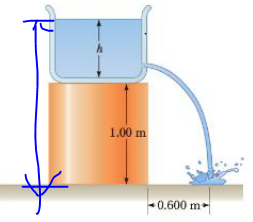Chapter 9, Problem 43P

Chapter
Section
Textbook Problem

A jet of water squirts out horizontally from a hole near the bottom of the tank shown in Figure P9.43. If the hole has a diameter of 3.50 mm, what is the height h of the water level in the tank?Figure P9.43

To determine
The height h of the water level in the tank.

Explanation
At first, the time for water to reach the ground by considering it as a projectile motion using Newton’s equations of motion is Δy=v0yt+(1/2)ayt2 with initial downward speed of water is v0y=0m/s and the height of the hole from the ground is 1.00m and the negative sign is because of the assumption of the hole is being at y=0m . The time is t=2(Δy)/ay . The horizontal speed of water is v2=v0x=Δx/t=Δx/2(Δy)/ay . Assume points 1 and 2 are assigned for top of the tank and for level of the hole. Both points have same pressure that is P1=P2=Patm and the speed of the water at top of the tank is v10m/s   Now Bernoulli’s equation is written for this problem as ρgy1=(1/2)ρv22+ρgy2y1y2=v22/2g

Still sussing out bartleby?

Check out a sample textbook solution.

See a sample solution

The Solution to Your Study Problems

Bartleby provides explanations to thousands of textbook problems written by our experts, many with advanced degrees!

Get Started

What are the stages of protein synthesis?

Introduction to General, Organic and Biochemistry

Why did the Copernican hypothesis win gradual acceptance?

Horizons: Exploring the Universe (MindTap Course List)

Define the following terms: a. chromosome b. chromatin

Human Heredity: Principles and Issues (MindTap Course List)

Define the term turnover number.

Chemistry for Today: General, Organic, and Biochemistry